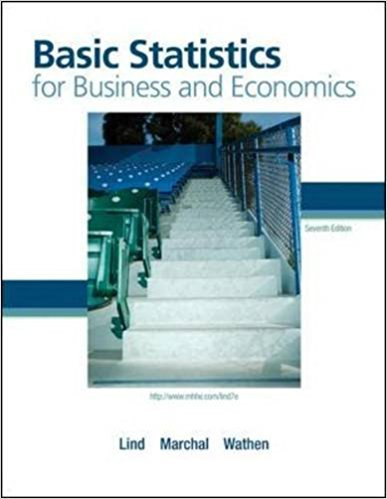×
×

# Let P(X) = .55 and P(Y) = .35. Assume the probability thatISBN: 9780077384470 64

## Solution for problem 18E Chapter 5

Basic Statistics for Business and Economics | 7th Edition

• Textbook Solutions
• 2901 Step-by-step solutions solved by professors and subject experts
• Get 24/7 help from StudySoup virtual teaching assistantsBasic Statistics for Business and Economics | 7th Edition

4 5 0 411 Reviews
17
0
Problem 18E

Let P(X) = .55 and P(Y) = .35. Assume the probability that they both occur is .20. What is the probability of either X or Y occurring?

Step-by-Step Solution:

Step 1 of 1</p>

We have to find the probability that either X or Y occurring

Given thatThe probability of both of them occurring is 0.2

That meansNow the probability...

Step 2 of 3

Step 3 of 3

##### ISBN: 9780077384470

Unlock Textbook Solution